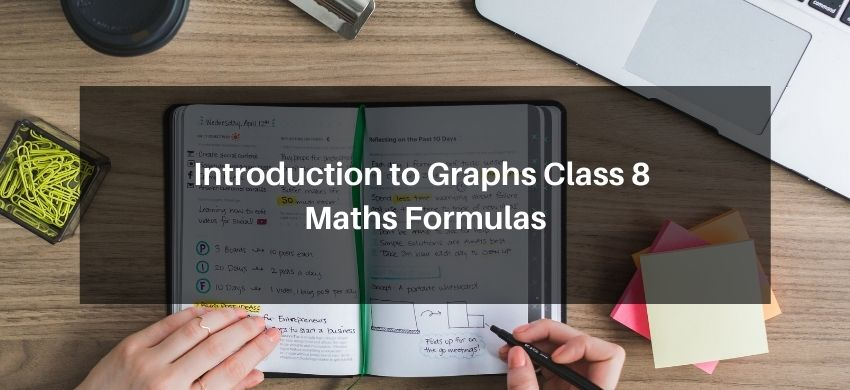# Introduction to Graphs Class 8 Maths FormulasHey, students are you looking for Introduction to Graphs Class 8 Maths Formulas? If yes. Then you are at the right place. In this post, I have listed all the formulas of Introduction to Graph class 8 that you can use to learn and understand the concepts easily.

If you want to improve your class 8 Math, Introduction to Graphs concepts, then it is super important for you to learn and understand all the formulas.

By using these formulas you will learn about Introduction to Graphs.

With the help of these formulas, you can revise the entire chapter easily.

## Introduction to Graphs Class 8 Maths Formulas

• The x-coordinate of a point is its distance from y-axis.
• The y-coordinate of a point is its distance from x-axis.
• The coordinates of the origin are (0, 0).
• The x-coordinate of every point on y-axis is zero.
• The y-coordinate of every point on x-axis is zero.
• A bar graph is used to show comparison among categories.
• A pie graph is used to compare parts of a whole.
• A histogram is a bar graph that shows data in intervals.
• A line graph displays data that changes continuously over periods of time.
• A line graph which is a whole unbroken line is called a linear graph.
• Fixing a point on the graph sheet we need, x-coordinate and y-coordinate.
• The relation between dependent variable and independent variable is shown through a graph.
• The purpose of the graph is to show numerical facts in visual form for their better and quick understanding. It is specially very useful when there is a trend or comparison to be shown.
• A Bar Graph consists of two or more parallel vertical (or horizontal) bars (rectangles).